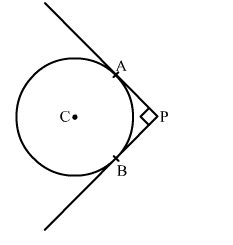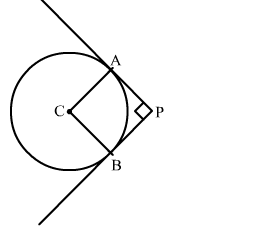# In the given figure, DE and DF are two tangents drawn from an external point D to a circle with centre A.`
Question:

In the given figure, DE and DF are two tangents drawn from an external point D to a circle with centre A. If DE = 5 cm. and  DE ⊥ DF then the radius of the circle is

(a) 3 cm
(b) 4 cm
(c) 5 cm
(d) 6 cmSolution:

Construction: Join AF and AEWe know that the radius and tangent are perperpendular at their point of contact
∵∠AED = ∠AFD = 90
Since, in quadrilateral AEDF all the angles are right angles
∴ AEDF is a rectangle
Now, we know that the pair of opposite sides are equal in rectangle
∴ AF = DE = 5 cm
Therefore, the radius of the circle is 5 cm
Hence, the correct answer is option (c).